# 数据结构与算法——计数排序

## 算法介绍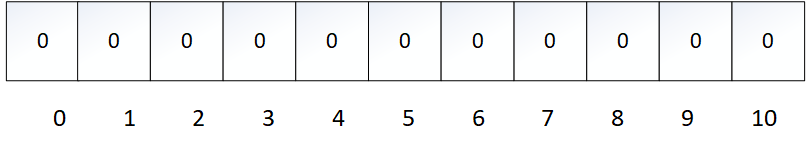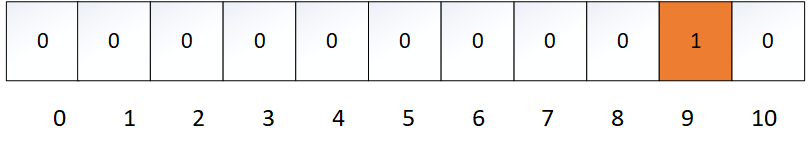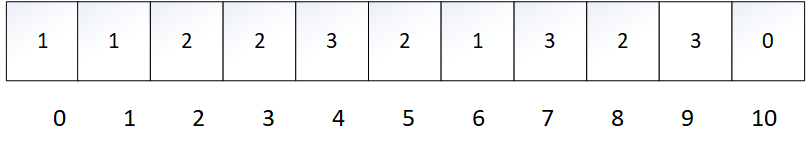## 算法实现

``````public static int[] sort(int[] arr) {
// 空数组直接返回
if (arr.length==0) return arr;
// 新建数组储存排序后的结果
int[] res = new int[arr.length];
// 得到数列的最大值
int max = arr;
for (int i: arr) {
if (max < i) max = i;
}
// 根据数列最大值确定统计数组的长度
int[] cntArr = new int[max+1];
// 遍历数组，填充统计数组
for (int i: arr) {
cntArr[i]++;
}
// 遍历统计数组,得到排好序后的数组
int index = 0;
for (int i=0; i<cntArr.length; i++) {
for (int j=0; j<cntArr[i]; j++) {
res[index++] = i;
}
}
return res;
}
``````

## 算法优化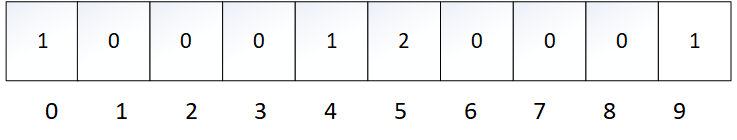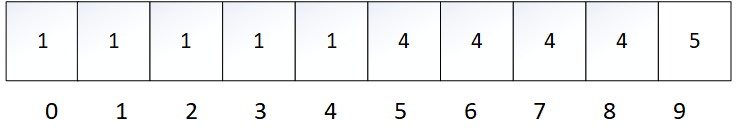``````public static int[] sort(int[] arr) {
// 空数组直接返回
if (arr.length==0) return arr;
// 新建数组储存排序后的结果
int[] res = new int[arr.length];
// 得到数列的最大值，最小值
int max = arr;
int min = max;
for (int i: arr) {
if (max < i) max = i;
if (min > i) min = i;
}
// 根据数列最大最小值确定统计数组的长度
int[] cntArr = new int[max-min+1];
// 遍历数组，填充统计数组
for (int i: arr) cntArr[i-min]++;
// 遍历统计数组累加计数
for (int i=1; i<cntArr.length; i++) cntArr[i]+=cntArr[i-1];
// 倒序遍历数组,得到排好序后的数组
for (int i=arr.length-1; i>=0; i--) res[--cntArr[arr[i]-min]] = arr[i];
return res;
}
``````# Hao JiangJustin Time

We only live once, and time just goes by.

•R&D Engineer @H3C
•Hangzhou, China